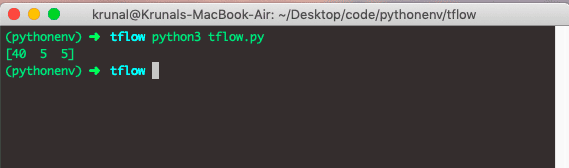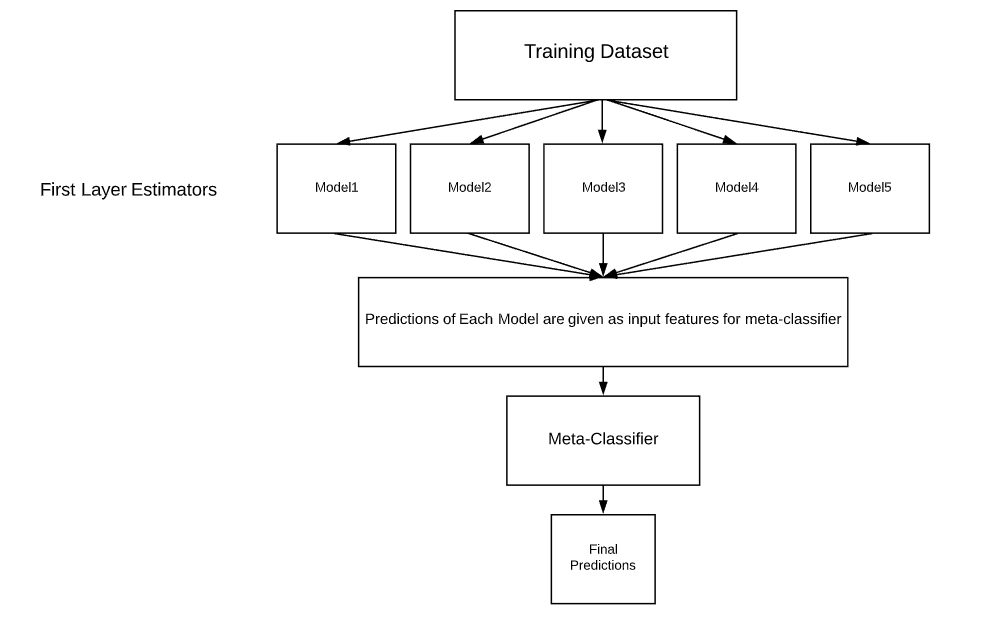1564894616

# How To Build Simple Model In Tensorflow

Tensorflow is created at Google. It is an open source machine learning framework for everyone. TensorFlow is an open source library for high-performance numerical computation. Tensorflow has a flexible architecture that allows easy deployment of calculation across a variety of platforms like CPUs, GPUs, TPUs, and from desktops to clusters of servers to mobile and edge devices. It is initially developed by researchers and engineers from the Google Brain team within Google’s AI organization, and it comes with strong support for machine learning and deep learning, and the flexible numerical computation core is used across many other scientific domains.

## How To Build Simple Model In Tensorflow

If we want to work with Tensorflow then first, we need to define the tensorflow graph. In this model, we will define two tensors or nodes, and then we add those nodes. Now, let’s dive into what is tensors.

## Tensors in Tensorflow

TensorFlow, as the name indicates, is the framework to define and run computations involving tensors. The tensor is the generalization of vectors and matrices to potentially higher dimensions. Internally, TensorFlow represents tensors as n-dimensional arrays of base datatypes.

When writing a TensorFlow program, the primary object you manipulate and pass around is the tf.Tensor. The **tf.Tensor **object represents a partially defined computation that will eventually produce a value. TensorFlow programs work by first building a graph of tf.Tensor objects, detailing how each tensor is computed based on the other available tensors and then by running parts of this graph to achieve the desired results.

TensorFlow programs use the tensor data structure to represent all data only tensors are passed between operations in the computation graph. You can think of the TensorFlow tensor as an n-dimensional array or list.

For example, a scaler is a tensor, a vector is a tensor, and a matrix is a tensor. A tensor has a rank, a shape, and a static type so that a tensor can be represented as the multidimensional array of numbers.

## Tensorflow Example

Okay, now, if you do not know how to install and configure the Tensorflow on virtualenv then check out my post on how to install tensorflow on this blog.

Okay, now we will create a file called **tflow.py **and write the following code inside that file.

``````# app.py

import tensorflow as tf
import os

os.environ['TF_CPP_MIN_LOG_LEVEL'] = '2'

X = tf.placeholder(tf.int32, name='X')
Y = tf.placeholder(tf.int32, name='Y')

with tf.Session() as session:
X: [19, 1, 2],
Y: [21, 4, 3]
})
print(result)

``````

In the above code, first, we have imported the **tensorflow **and **os module **from the Python library.

We have used the **os **module’s **environ attribute **to disable the logs which are unnecessary for this demo model. If you want that log in the output, then you can comment that statement.

Then we have created the computational graph with two nodes using **a placeholder **and defined their datatypes and names.

So, we have two tensors up to now which is X and Y.

Then we are adding those tensors using tensorflow’s add method and get the third node which is an add node.

If we want to execute operation in the Graph, we need to use sessions.

We can create a session in tensorflow using **with tf.Session as session **code.

Next step is that we have called the run method on the session, which takes the two arguments.

2. data

We have used the dictionary data, which has two nodes, X and Y. So we will add those and generate a new node with the session.

Go to the terminal and run the following command.

``````python3 tflow.py

``````

The output of the above code is following.So, we have built a tensorflow model, which can add two nodes and gives the output node.

Here, the computation is to add the nodes and nothing complicated. But in real life application, there are lots of variables and iterables to go through and finally predict the future value.

Finally, How To Build Simple Model In Tensorflow Tutorial With Example is over.

#tensorflow #python #machine-learning

## Buddha Community1564928324

Thanks !1601993220

## How To Build Simple Model In Tensorflow

In this tutorial, I will show you How To Build Simple Model In Tensorflow. Tensorflow Framework is the popular framework to design a neural network in Machine Learning. Tensorflow is created at Google. It is an open source machine learning framework for everyone. TensorFlow is an open source library for high-performance numerical computation. Tensorflow has a flexible architecture that allows easy deployment of calculation across a variety of platforms like CPUs, GPUs, TPUs, and from desktops to clusters of servers to mobile and edge devices. It is initially developed by researchers and engineers from the Google Brain team within Google’s AI organization, and it comes with strong support for machine learning and deep learning, and the flexible numerical computation core is used across many other scientific domains.

### How To Build Simple Model In Tensorflow

If we want to work with Tensorflow then first, we need to define the tensorflow graph. In this model, we will define two tensors or nodes, and then we add those nodes. Now, let’s dive into what is tensors.

#tensorflow #tensorflow framework #machine learning1621931885

## How TensorFlow Lite Fits In The TinyML Ecosystem

TensorFlow Lite has emerged as a popular platform for running machine learning models on the edge. A microcontroller is a tiny low-cost device to perform the specific tasks of embedded systems.

In a workshop held as part of Google I/O, TensorFlow founding member Pete Warden delved deep into the potential use cases of TensorFlow Lite for microcontrollers.

Further, quoting the definition of TinyML from a blog, he said:

“Tiny machine learning is capable of performing on-device sensor data analytics at extremely low power, typically in the mW range and below, and hence enabling a variety of ways-on-use-case and targeting battery operated devices.”

#opinions #how to design tinyml #learn tinyml #machine learning models low cost #machine learning models low power #microcontrollers #tensoflow latest #tensorflow lite microcontrollers #tensorflow tinyml #tinyml applications #tinyml models1623906928

## What is Model Stacking?

Model Stacking is a way to improve model predictions by combining the outputs of multiple models and running them through another machine learning model called a meta-learner. It is a popular strategy used to win kaggle competitions, but despite their usefulness they’re rarely talked about in data science articles — which I hope to change.

Essentially a stacked model works by running the output of multiple models through a “meta-learner” (usually a linear regressor/classifier, but can be other models like decision trees). The meta-learner attempts to minimize the weakness and maximize the strengths of every individual model. The result is usually a very robust model that generalizes well on unseen data.

The architecture for a stacked model can be illustrated by the image below:#tensorflow #neural-networks #model-stacking #how to use “model stacking” to improve machine learning predictions #model stacking #machine learning1624653660

## The Best Way to Build a Chatbot in 2021

A useful tool several businesses implement for answering questions that potential customers may have is a chatbot. Many programming languages give web designers several ways on how to make a chatbot for their websites. They are capable of answering basic questions for visitors and offer innovation for businesses.

With the help of programming languages, it is possible to create a chatbot from the ground up to satisfy someone’s needs.

### Plan Out the Chatbot’s Purpose

Before building a chatbot, it is ideal for web designers to determine how it will function on a website. Several chatbot duties center around fulfilling customers’ needs and questions or compiling and optimizing data via transactions.

Some benefits of implementing chatbots include:

• Generating leads for marketing products and services
• Improve work capacity when employees cannot answer questions or during non-business hours
• Reducing errors while providing accurate information to customers or visitors
• Meeting customer demands through instant communication

Some programmers may choose to design a chatbox to function through predefined answers based on the questions customers may input or function by adapting and learning via human input.

#chatbots #latest news #the best way to build a chatbot in 2021 #build #build a chatbot #best way to build a chatbot1619255340

## Simple TensorFlow Model To Predict a Linear Regression

### Introduction

In this article, we will learn to create a simple TensorFlow model to predict value based on the linear regression equation.

Steps To Build the Model

Conclusion

Popular on DZone

#machine learning #data science #artifical intelligence #tensorflow #linear regression #tensorflow tutorial